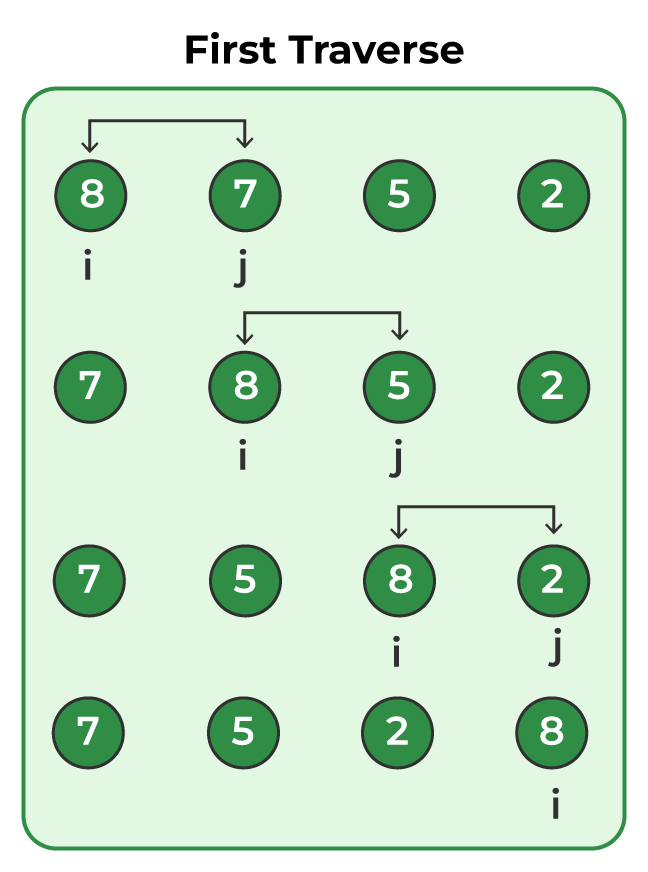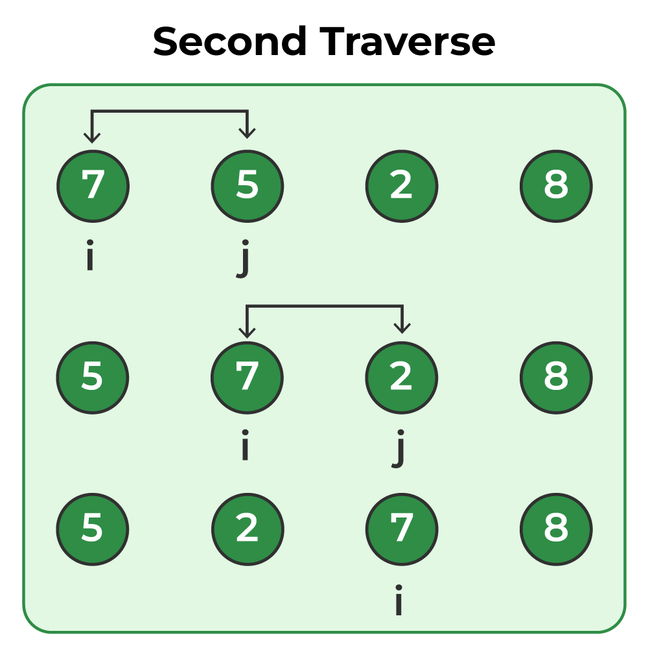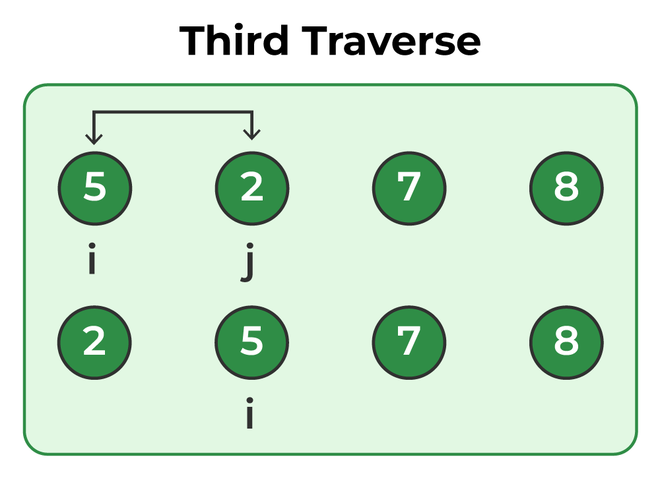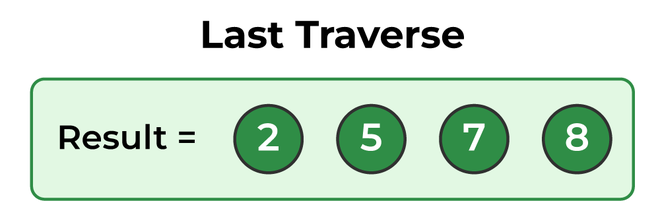Open In App

# Java Program for Bubble Sort

Bubble Sort is the simplest sorting algorithm that works by repeatedly swapping the adjacent elements if they are in the wrong order. Bubble Sort in Java is not the best method to sort an array but is one of the most basic implementations for one to learn. In this article, we will learn how to write a program for Bubble Sort in Java.

## Algorithm for Bubble Sort in Java

The following is the algorithm to sort array in increasing order using bubble sort in Java:

1. Start
2. Initiate two values n as size of array ,also i and j to traverse array.
3. Put i=0 and j=1.
4. While traversing if array[i] > array[j] swap both the numbers.
5. Increment the value i and j then goto Step 3.
6. If the value of i > n-1 and j > n and n>1 then
• n=n-1
• goto Step 2
7. Exit

## Example of Bubble Sort

### Step1:

Given, array: [ 8,7,5,2] , traverse from pos=1 till pos=4 and swap the number with the previous element if it is less than the previous element.### Step 2:

Repeat the previous Step for pos=1 to pos=3### Step 3:

Follow Step 1 for pos=1 to pos=2### Step 4:

As now pos=1 to pos=1 means the array is now fully sorted## Program for Bubble Sort in Java

Below is the Program implementing Bubble Sort in Java

## Java

 `// Java program for implementation``// of Bubble Sort``class` `BubbleSort {``    ``void` `bubbleSort(``int` `arr[])``    ``{``        ``int` `n = arr.length;``        ``for` `(``int` `i = ``0``; i < n - ``1``; i++)``            ``for` `(``int` `j = ``0``; j < n - i - ``1``; j++)``                ``if` `(arr[j] > arr[j + ``1``]) {``                    ``// swap temp and arr[i]``                    ``int` `temp = arr[j];``                    ``arr[j] = arr[j + ``1``];``                    ``arr[j + ``1``] = temp;``                ``}``    ``}`` ` `    ``// Prints the array``    ``void` `printArray(``int` `arr[])``    ``{``        ``int` `n = arr.length;``        ``for` `(``int` `i = ``0``; i < n; ++i)``            ``System.out.print(arr[i] + ``" "``);``        ``System.out.println();``    ``}`` ` `    ``// Driver method to test above``    ``public` `static` `void` `main(String args[])``    ``{``        ``BubbleSort ob = ``new` `BubbleSort();``        ``int` `arr[] = { ``64``, ``34``, ``25``, ``12``, ``22``, ``11``, ``90` `};``        ``ob.bubbleSort(arr);``        ``System.out.println(``"Sorted array"``);``        ``ob.printArray(arr);``    ``}``}`

Output

```Sorted array
11 12 22 25 34 64 90 ```

### Complexity Analysis of Java Bubble Sort

Time Complexity: O(n2)
Auxiliary Space: O(1)

### Advantage of Bubble Sort in Java

Despite Bubble Sort Being a bit slow it has certain advantages too as mentioned below:

• Easy to implement and understand.
• The space Complexity of the algorithm is O(1) as no extra memory is required.
• It is a stable sorting algorithm, meaning that elements with the same key value maintain their relative order in the sorted output.

### Reference Articles

Please refer complete article on Bubble Sort for more details!